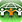GMAT Question of the Day - Daily to your Mailbox; hard ones only

 It is currently 20 Aug 2019, 12:38### GMAT Club Daily Prep

#### Thank you for using the timer - this advanced tool can estimate your performance and suggest more practice questions. We have subscribed you to Daily Prep Questions via email.

Customized
for You

we will pick new questions that match your level based on your Timer History

Track
Your Progress

every week, we’ll send you an estimated GMAT score based on your performance

Practice
Pays

we will pick new questions that match your level based on your Timer History

#### Not interested in getting valuable practice questions and articles delivered to your email? No problem, unsubscribe here.### Request Expert Reply# In the interior of a forest, a certain number of apes equal to the squ

 new topic post reply Question banks Downloads My Bookmarks Reviews Important topics
Author Message
TAGS:

### Hide Tags

ManagerJoined: 30 Sep 2010
Posts: 51
In the interior of a forest, a certain number of apes equal to the squ  [#permalink]

### Show Tags

500:00

Difficulty:65% (hard)

Question Stats:70% (03:13) correct30% (02:38) wrongbased on 209 sessions

### HideShow timer Statistics

In the interior of a forest, a certain number of apes equal to the square of one-eighth of the total number are playing and having great fun. The remaining twelve apes are on a hill and the echo of their shrieks by the adjoining hills frightens them. They came and join the apes in the forest and play with enthusiasm. What is the total number of apes?

A. 48
B. 16
C. 64
D. 80
E. 16 or 48
InternB
Joined: 15 Aug 2017
Posts: 13
In the interior of a forest, a certain number of apes equal to the squ  [#permalink]

### Show Tags

3
Verifying answer options with the given condintion,
Take 1 st option If total apes 48
apes interior= total-exterior= 48-12=36
apes interior= square of {(1/8)th of total(48)}= 6^2 =36.
So 48 is a possible solution.
So either A or E.
When checking with 16 we can find that it satisfies too.
So E
KudosRetired ModeratorJoined: 02 Sep 2010
Posts: 755
Location: London
Re: In the interior of a forest, a certain number of apes equal to the squ  [#permalink]

### Show Tags

Let total number be x
No in the interior = (x/8)^2
No outside = 12
So : x - (x/8)^2=12
x^2-64x+12*64=0
x^2-16x-48x+16*48=0
(x-16)(x-48)=0

So either x=16 or x=48Posted from GMAT ToolKit
_________________
SVPStatus: The Best Or Nothing
Joined: 27 Dec 2012
Posts: 1785
Location: India
Concentration: General Management, Technology
WE: Information Technology (Computer Software)
Re: In the interior of a forest, a certain number of apes equal to the squ  [#permalink]

### Show Tags

2
Let total no of apes = x

Square of $$\frac{1}{8} th$$ are playing $$= (\frac{x}{8})^2 = \frac{x^2}{64}$$

Remaining = 12

Setting up quadratic equation

$$x = \frac{x^2}{64} + 12$$

$$x^2 - 64x + 12*64 = 0$$

This equation will not have -ve value of x as middle term is -ve & last term is +ve; This will have two answers

In the OA, only option E has two answers

Answer = E
_________________
Kindly press "+1 Kudos" to appreciateSenior ManagerP
Joined: 02 Apr 2014
Posts: 471
Location: India
Schools: XLRI"20
GMAT 1: 700 Q50 V34GPA: 3.5
Re: In the interior of a forest, a certain number of apes equal to the squ  [#permalink]

### Show Tags

1
Let total number of apes = 8x
number of playing = $$x^2$$
remaining = 12

given: $$x^2 + 12 = 8x$$ => $$x^2 - 8x + 12 = 0$$=> x = 2 or 6
so total number of apes = 16 or 48 (E)Re: In the interior of a forest, a certain number of apes equal to the squ   [#permalink] 24 Dec 2017, 07:11
Display posts from previous: Sort by

# In the interior of a forest, a certain number of apes equal to the squ

 new topic post reply Question banks Downloads My Bookmarks Reviews Important topics

 Powered by phpBB © phpBB Group | Emoji artwork provided by EmojiOne

#### MBA Resources